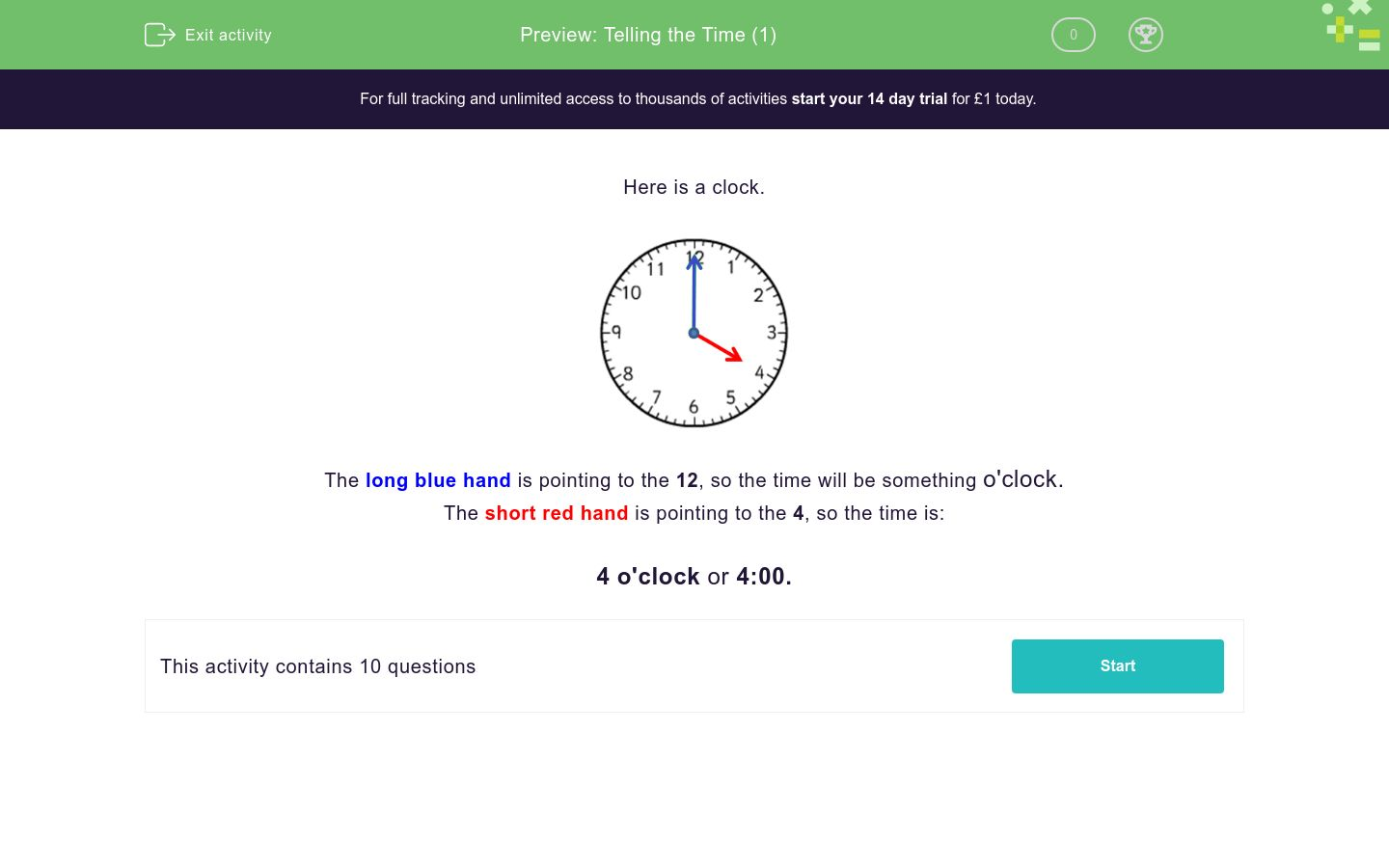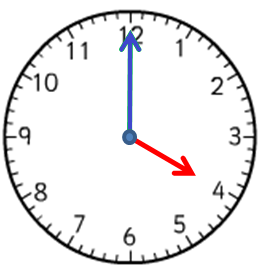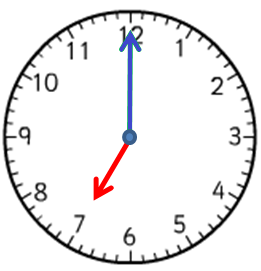# Telling the Time (1)

In this worksheet, students must select the time that matches the given clock face. Only o'clock times are shown.Key stage:  KS 1

Curriculum topic:   Measurement

Curriculum subtopic:   Tell the Time

Difficulty level:### QUESTION 1 of 10

Here is a clock.The long blue hand is pointing to the 12, so the time will be something o'clock.

The short red hand is pointing to the 4, so the time is:

4 o'clock or 4:00.

Which time is shown on the clock?12 o'clock

2 o'clock

10 o'clock

Which time is shown on the clock?3 o'clock

12 o'clock

9 o'clock

Which time is shown on the clock?12:00

5:00

7:00

Which time is shown on the clock?3:00

5:00

12:00

Which time is shown on the clock?3:00

6:00

12:00

Write the time shown on the clock.

Use a colon : to separate the hour from the minutes (for example, 7:00).Write the time shown on the clock.

Use a colon : to separate the hour from the minutes (for example, 7:00).Write the time shown on the clock.

Use a colon : to separate the hour from the minutes (for example, 7:00).Write the time shown on the clock.

Use a colon : to separate the hour from the minutes (for example, 7:00).Write the time shown on the clock.

Use a colon : to separate the hour from the minutes (for example, 7:00).• Question 1

Which time is shown on the clock?2 o'clock
EDDIE SAYS
The short hand is pointing to 2.
The long hand is pointing to 12.
• Question 2

Which time is shown on the clock?9 o'clock
EDDIE SAYS
The short hand is pointing to 9.
The long hand is pointing to 12.
• Question 3

Which time is shown on the clock?7:00
EDDIE SAYS
The short hand is pointing to 7.
The long hand is pointing to 12.
• Question 4

Which time is shown on the clock?3:00
EDDIE SAYS
The short hand is pointing to 3.
The long hand is pointing to 12.
• Question 5

Which time is shown on the clock?6:00
EDDIE SAYS
The short hand is pointing to 6.
The long hand is pointing to 12.
• Question 6

Write the time shown on the clock.

Use a colon : to separate the hour from the minutes (for example, 7:00).11:00
EDDIE SAYS
The short hand is pointing to 11.
The long hand is pointing to 12.
• Question 7

Write the time shown on the clock.

Use a colon : to separate the hour from the minutes (for example, 7:00).05:00
5:00
EDDIE SAYS
The short hand is pointing to 5.
The long hand is pointing to 12.
• Question 8

Write the time shown on the clock.

Use a colon : to separate the hour from the minutes (for example, 7:00).08:00
8:00
EDDIE SAYS
The short hand is pointing to 8.
The long hand is pointing to 12.
• Question 9

Write the time shown on the clock.

Use a colon : to separate the hour from the minutes (for example, 7:00).01:00
1:00
EDDIE SAYS
The short hand is pointing to 1.
The long hand is pointing to 12.
• Question 10

Write the time shown on the clock.

Use a colon : to separate the hour from the minutes (for example, 7:00).10:00
EDDIE SAYS
The short hand is pointing to 10.
The long hand is pointing to 12.
---- OR ----

Sign up for a £1 trial so you can track and measure your child's progress on this activity.

### What is EdPlace?

We're your National Curriculum aligned online education content provider helping each child succeed in English, maths and science from year 1 to GCSE. With an EdPlace account you’ll be able to track and measure progress, helping each child achieve their best. We build confidence and attainment by personalising each child’s learning at a level that suits them.

Get started